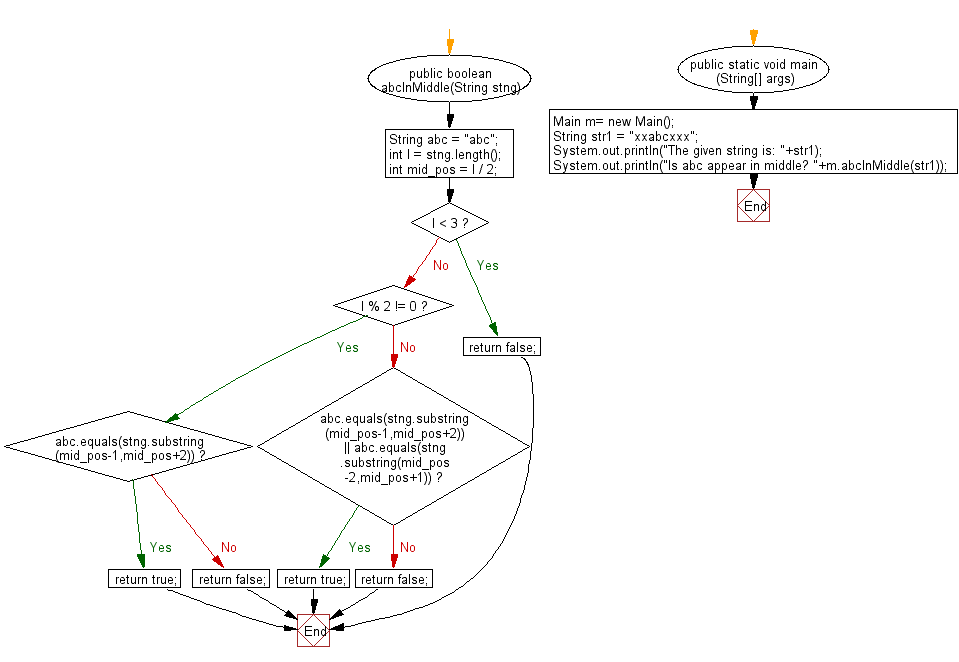﻿ Java - Check if a substring presents in the middle of another

# Java: Check whether a substring presents in the middle of another string

## Java String: Exercise-75 with Solution

Write a Java program to check whether a given substring appears in the middle of another string. Here middle means the difference between the number of characters to the left and right of the given substring is not more than 1.

Visual Presentation:Sample Solution:

Java Code:

``````import java.util.*;

// Define a class named Main
public class Main {

// Method to check if "abc" appears in the middle of the string
public boolean abcInMiddle(String stng) {
String abc = "abc"; // Define the substring to be checked
int l = stng.length(); // Get the length of the input string
int mid_pos = l / 2; // Calculate the middle position of the string

if (l < 3)
return false; // If the length is less than 3, "abc" cannot appear in the middle

if (l % 2 != 0) { // If the length is odd
if (abc.equals(stng.substring(mid_pos - 1, mid_pos + 2))) {
return true; // If the substring from mid-1 to mid+1 matches "abc", return true
} else {
return false; // If not, return false
}
} else if (abc.equals(stng.substring(mid_pos - 1, mid_pos + 2)) || abc.equals(stng.substring(mid_pos - 2, mid_pos + 1))) {
return true; // If the substring from mid-1 to mid+1 or mid-2 to mid matches "abc", return true
} else {
return false; // If not, return false
}
}

// Main method to execute the program
public static void main(String[] args) {
Main m = new Main(); // Create an instance of the Main class

String str1 = "xxabcxxx"; // Input string to be checked
System.out.println("The given string is: " + str1);
System.out.println("Does 'abc' appear in the middle? " + m.abcInMiddle(str1)); // Display the result
}
}
``````

Sample Output:

```The given string is: xxxabcxxxxx
Is abc appear in middle? false

The given string is: xxabcxxx
Is abc appear in middle? true
```

Flowchart:Java Code Editor:

Improve this sample solution and post your code through Disqus

What is the difficulty level of this exercise?

Test your Programming skills with w3resource's quiz.

﻿